The PANEL Procedure

Parks Method (Autoregressive Model)

Subsections:

Parks (1967) considered the first-order autoregressive model in which the random errors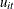,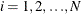, and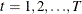have the structure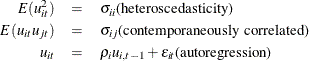where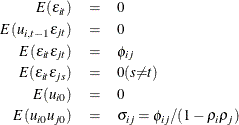The model assumed is first-order autoregressive with contemporaneous correlation between cross sections. In this model, the covariance matrix for the vector of random errors u can be expressed as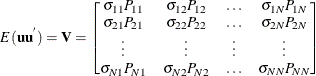where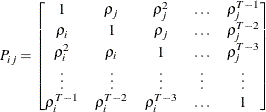The matrix V is estimated by a two-stage procedure, and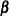is then estimated by generalized least squares. The first step in estimating V involves the use of ordinary least squares to estimateand obtain the fitted residuals, as follows: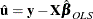A consistent estimator of the first-order autoregressive parameter is then obtained in the usual manner, as follows: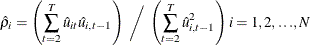Finally, the autoregressive characteristic of the data is removed (asymptotically) by the usual transformation of taking weighted differences. That is, for,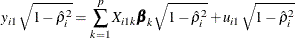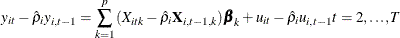which is written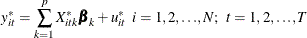Notice that the transformed model has not lost any observations (Seely and Zyskind, 1971).

The second step in estimating the covariance matrix V is applying ordinary least squares to the preceding transformed model, obtaining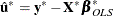from which the consistent estimator of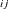is calculated as follows: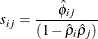where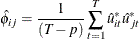Estimated generalized least squares (EGLS) then proceeds in the usual manner,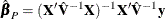where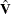is the derived consistent estimator of V. For computational purposes,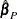is obtained directly from the transformed model,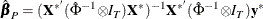where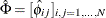.

The preceding procedure is equivalent to Zellner’s two-stage methodology applied to the transformed model (Zellner, 1962).

Parks demonstrates that this estimator is consistent and asymptotically, normally distributed with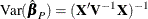Standard Corrections

For the PARKS option, the first-order autocorrelation coefficient must be estimated for each cross section. Let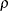be the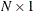vector of true parameters and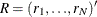be the corresponding vector of estimates. Then, to ensure that only range-preserving estimates are used in PROC PANEL, the following modification for R is made: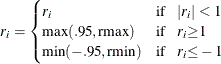where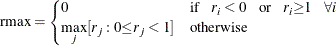and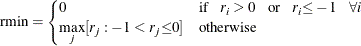Whenever this correction is made, a warning message is printed.